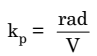# Mathematical expression of Single-tone Phase Modulated Wave

### Mathematical expression of Single-tone Phase Modulated Wave

As we already knew, phase modulation is another type of angle modulation. The PM wave is obtained by varying the phase angle Φ of a carrier in proportion with the amplitude of the modulating voltage.

If the carrier voltage is expressed as under: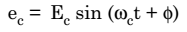Then, the PM wave can be expressed as under: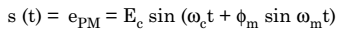Here, Φm = Maximum phase change corresponding to the maximum amplitude of the modulating signal.

For the sake of uniformity, let us modify the above equation  as under: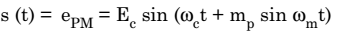where, mp = Φm = Modulation index of PM = phase deviation.

The FM and PM waves look identical when their modulation index are identical. However, if we change the modulating frequency fm, then mf will change but there is no change in the value of mp.

### Deviation Sensitivity of PM

For the modulating signal :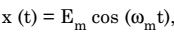the instantaneous phase deviation is given by,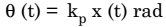Where,kp is the deviation sensitivity of phase modulation.

Thus,